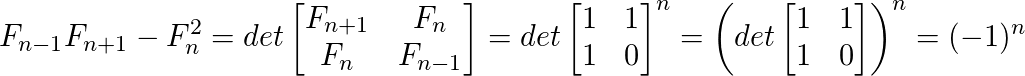# Cassini’s Identity

Given a number N, the task is to evaluate below expression. Expected time complexity is O(1).

` f(n-1)*f(n+1) - f(n)*f(n)`

Where f(n) is the n-th Fibonacci number with n >= 1. First few Fibonacci numbers are 0, 1, 1, 2, 3, 5, 8, 13, ………..i.e. (considering 0 as 0th Fibonacci number)

Examples :

```Input : n = 5
Output : -1
f(5-1=4) = 3
f(5+1=6) = 8
f(5)*f(5)= 5*5 = 25
f(4)*f(6)- f(5)*f(5)= 24-25= -1
```

## Recommended: Please solve it on “PRACTICE ” first, before moving on to the solution.

Although the task is simple i.e. find n-1th, nth and (n+1)-th Fibonacci numbers. Evaluate the expression and display the result. But this can be done in O(1) time using Cassini’s Identity which states that:

`           f(n-1)*f(n+1) - f(n*n) = (-1)^n `

So, we don’t need to calculate any Fibonacci term,the only thing is to check whether n is even or odd.

How does above formula work?
The formula is based on matrix representation of Fibonacci numbers.## C/C++

 `// C++ implementation to demonstrate working ` `// of Cassini’s Identity  ` `#include ` `using` `namespace` `std; ` ` `  `// Returns (-1)^n ` `int` `cassini(``int` `n) ` `{ ` `   ``return` `(n & 1) ? -1 : 1; ` `}  ` ` `  `// Driver program ` `int` `main() ` `{   ` `   ``int` `n = 5; ` `   ``cout << cassini(n); ` `   ``return` `0; ` `}  `

## Java

 `// Java implementation to demonstrate working ` `// of Cassini’s Identity  ` ` `  `class` `Gfg ` `{ ` `    ``// Returns (-1)^n ` `    ``static` `int` `cassini(``int` `n) ` `    ``{ ` `       ``return` `(n & ``1``) != ``0` `? -``1` `: ``1``; ` `    ``}  ` ` `  `    ``// Driver method ` `    ``public` `static` `void` `main(String args[]) ` `    ``{ ` `         ``int` `n = ``5``; ` `         ``System.out.println(cassini(n)); ` `    ``} ` `} `

## Python3

 `# Python implementation ` `# to demonstrate working ` `# of Cassini’s Identity  ` ` `  `# Returns (-1)^n ` `def` `cassini(n): ` ` `  `   ``return` `-``1` `if` `(n & ``1``) ``else` `1` `  `  `# Driver program ` `  `  `n ``=` `5` `print``(cassini(n)) ` `    `  `# This code is contributed ` `# by Anant Agarwal. `

## C#

 `// C# implementation to demonstrate  ` `// working of Cassini’s Identity ` `using` `System; ` ` `  `class` `GFG { ` ` `  `    ``// Returns (-1) ^ n ` `    ``static` `int` `cassini(``int` `n) ` `    ``{ ` `       ``return` `(n & 1) != 0 ? -1 : 1; ` `    ``}  ` `  `  `    ``// Driver Code ` `    ``public` `static` `void` `Main() ` `    ``{ ` `         ``int` `n = 5; ` `         ``Console.Write(cassini(n)); ` `    ``} ` `} ` ` `  `// This code is contributed by Nitin Mittal. `

## PHP

 ` `

Output :

```-1
```

This article is contributed by Sahil Chhabra. If you like GeeksforGeeks and would like to contribute, you can also write an article using contribute.geeksforgeeks.org or mail your article to contribute@geeksforgeeks.org. See your article appearing on the GeeksforGeeks main page and help other Geeks.

Attention reader! Don’t stop learning now. Get hold of all the important DSA concepts with the DSA Self Paced Course at a student-friendly price and become industry ready.

My Personal Notes arrow_drop_up

Improved By : nitin mittal, jit_t

Article Tags :
Practice Tags :

Be the First to upvote.

Please write to us at contribute@geeksforgeeks.org to report any issue with the above content.